Download Presentation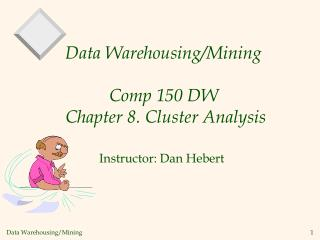Data Warehousing/Mining Comp 150 DW Chapter 8. Cluster Analysis

# Data Warehousing/Mining Comp 150 DW Chapter 8. Cluster Analysis - PowerPoint PPT Presentation

Data Warehousing/Mining Comp 150 DW Chapter 8. Cluster Analysis. Instructor: Dan Hebert. Chapter 8. Cluster Analysis. What is Cluster Analysis? Types of Data in Cluster Analysis A Categorization of Major Clustering Methods Partitioning Methods Hierarchical Methods Density-Based MethodsI am the owner, or an agent authorized to act on behalf of the owner, of the copyrighted work described.
Download Presentation## Data Warehousing/Mining Comp 150 DW Chapter 8. Cluster Analysis

An Image/Link below is provided (as is) to download presentation

Download Policy: Content on the Website is provided to you AS IS for your information and personal use and may not be sold / licensed / shared on other websites without getting consent from its author.While downloading, if for some reason you are not able to download a presentation, the publisher may have deleted the file from their server.

- - - - - - - - - - - - - - - - - - - - - - - - - - E N D - - - - - - - - - - - - - - - - - - - - - - - - - -
Presentation Transcript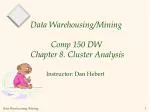### Data Warehousing/MiningComp 150 DW Chapter 8. Cluster Analysis

Instructor: Dan Hebert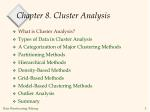Chapter 8. Cluster Analysis
• What is Cluster Analysis?
• Types of Data in Cluster Analysis
• A Categorization of Major Clustering Methods
• Partitioning Methods
• Hierarchical Methods
• Density-Based Methods
• Grid-Based Methods
• Model-Based Clustering Methods
• Outlier Analysis
• Summary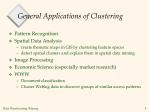General Applications of Clustering
• Pattern Recognition
• Spatial Data Analysis
• create thematic maps in GIS by clustering feature spaces
• detect spatial clusters and explain them in spatial data mining
• Image Processing
• Economic Science (especially market research)
• WWW
• Document classification
• Cluster Weblog data to discover groups of similar access patterns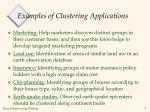Examples of Clustering Applications
• Marketing: Help marketers discover distinct groups in their customer bases, and then use this knowledge to develop targeted marketing programs
• Land use: Identification of areas of similar land use in an earth observation database
• Insurance: Identifying groups of motor insurance policy holders with a high average claim cost
• City-planning: Identifying groups of houses according to their house type, value, and geographical location
• Earth-quake studies: Observed earth quake epicenters should be clustered along continent faults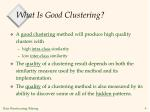What Is Good Clustering?
• A good clustering method will produce high quality clusters with
• high intra-class similarity
• low inter-class similarity
• The quality of a clustering result depends on both the similarity measure used by the method and its implementation.
• The quality of a clustering method is also measured by its ability to discover some or all of the hidden patterns.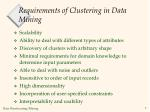Requirements of Clustering in Data Mining
• Scalability
• Ability to deal with different types of attributes
• Discovery of clusters with arbitrary shape
• Minimal requirements for domain knowledge to determine input parameters
• Able to deal with noise and outliers
• Insensitive to order of input records
• High dimensionality
• Incorporation of user-specified constraints
• Interpretability and usability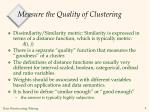Measure the Quality of Clustering
• Dissimilarity/Similarity metric: Similarity is expressed in terms of a distance function, which is typically metric: d(i, j)
• There is a separate “quality” function that measures the “goodness” of a cluster.
• The definitions of distance functions are usually very different for interval-scaled, boolean, categorical, ordinal and ratio variables.
• Weights should be associated with different variables based on applications and data semantics.
• It is hard to define “similar enough” or “good enough”
• the answer is typically highly subjective.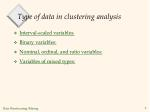Type of data in clustering analysis
• Interval-scaled variables:
• Binary variables:
• Nominal, ordinal, and ratio variables:
• Variables of mixed types: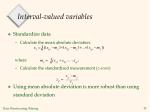Interval-valued variables
• Standardize data
• Calculate the mean absolute deviation:

where

• Calculate the standardized measurement (z-score)
• Using mean absolute deviation is more robust than using standard deviation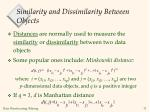Similarity and Dissimilarity Between Objects
• Distances are normally used to measure the similarity or dissimilarity between two data objects
• Some popular ones include: Minkowski distance:

where i = (xi1, xi2, …, xip) and j = (xj1, xj2, …, xjp) are two p-dimensional data objects, and q is a positive integer

• If q = 1, d is Manhattan distance

1/q

q

q

q

=

-

+

-

+

+

-

d

(

i

,

j

)

(|

x

x

|

|

x

x

|

...

|

x

x

|

)

i

j

i

j

i

j

1

1

2

2

p

pSimilarity and Dissimilarity Between Objects (Cont.)
• If q = 2, d is Euclidean distance:
• Properties
• d(i,j) 0
• d(i,i)= 0
• d(i,j)= d(j,i)
• d(i,j) d(i,k)+ d(k,j)Binary Variables
• 2 states – symmetric if no preference for which is 0 and 1
• A contingency table for binary data
• Simple matching coefficient (invariant, if the binary variable is symmetric):
• Jaccard coefficient (noninvariant if the binary variable is asymmetric)- ignore negative matches:

Object j

a - i & j both equal 1

b - i=1, j=0

Object iDissimilarity between Binary Variables
• Example – do patients have the same disease
• gender is a symmetric attribute (use Jaccard coefficeint so ignore )
• the remaining attributes are asymmetric binary
• let the values Y and P be set to 1, and the value N be set to 0

Most likely to have same disease

Unlikely to have same diseaseNominal Variables
• A generalization of the binary variable in that it can take more than 2 states, e.g., red, yellow, blue, green
• Method 1: Simple matching
• m: # of matches, p: total # of variables
• Method 2: use a large number of binary variables
• creating a new binary variable for each of the M nominal statesOrdinal Variables
• An ordinal variable can be discrete or continuous
• order is important, e.g., rank (gold, silver, bronze)
• Can be treated like interval-scaled-use following steps
• f is variable from set of variables, value of f for ith object is xif
• replace each xif by its’ rank
• map the range of each variable onto [0, 1] by replacing i-th object in the f-th variable by
• compute the dissimilarity using methods for interval-scaled variablesRatio-Scaled Variables
• Ratio-scaled variable: a positive measurement on a nonlinear scale, approximately at exponential scale, such as AeBt or Ae-Bt (A & B are positive constants)
• Methods:
• treat them like interval-scaled variables — not a good choice! Scale distorted
• apply logarithmic transformation

yif = log(xif)

• treat them as continuous ordinal data treat their rank as interval-scaled.Variables of Mixed Types
• A database may contain all the six types of variables
• symmetric binary, asymmetric binary, nominal, ordinal, interval and ratio.
• One may use a weighted formula to combine their effects.
• f is binary or nominal:

dij(f) = 0 if xif = xjf , or dij(f) = 1 o.w.

• f is interval-based: use the normalized distance
• f is ordinal or ratio-scaled
• compute ranks rif and
• and treat zif as interval-scaledMajor Clustering Approaches
• Partitioning algorithms: Construct various partitions and then evaluate them by some criterion
• Hierarchy algorithms: Create a hierarchical decomposition of the set of data (or objects) using some criterion
• Density-based: based on connectivity and density functions
• Grid-based: based on a multiple-level granularity structure
• Model-based: A model is hypothesized for each of the clusters and the idea is to find the best fit of that model to each otherPartitioning Algorithms: Basic Concept
• Partitioning method: Construct a partition of a database D of n objects into a set of k clusters
• Given a k, find a partition of k clusters that optimizes the chosen partitioning criterion
• Global optimal: exhaustively enumerate all partitions
• Heuristic methods: k-means and k-medoids algorithms
• k-means (MacQueen’67): Each cluster is represented by the center of the cluster
• k-medoids or PAM (Partition around medoids) (Kaufman & Rousseeuw’87): Each cluster is represented by one of the objects in the clusterThe K-Means Clustering Method
• Given k, the k-means algorithm is implemented in 4 steps:
• Partition objects into k nonempty subsets
• Compute seed points as the centroids of the clusters of the current partition. The centroid is the center (mean point) of the cluster.
• Assign each object to the cluster with the nearest seed point.
• Go back to Step 2, stop when no more new assignment.K-Means example
• 2, 3, 6, 8, 9, 12, 15, 18, 22 – break into 3 clusters
• Cluster 1 - 2, 8, 15 – mean = 8.3
• Cluster 2 - 3, 9, 18 – mean = 10
• Cluster 3 - 6, 12, 22 – mean = 13.3
• Re-assign
• Cluster 1 - 2, 3, 6, 8, 9 – mean = 5.6
• Cluster 2 – mean = 0
• Cluster 3 – 12, 15, 18, 22 – mean = 16.75
• Re-assign
• Cluster 1 – 3, 6, 8, 9 – mean = 6.5
• Cluster 2 – 2 – mean = 2
• Cluster 3 = 12, 15, 18, 22 – mean = 16.75K-Means example (continued)
• Re-assign
• Cluster 1 - 6, 8, 9 – mean = 7.6
• Cluster 2 – 2, 3 – mean = 2.5
• Cluster 3 – 12, 15, 18, 22 – mean = 16.75
• Re-assign
• Cluster 1 - 6, 8, 9 – mean = 7.6
• Cluster 2 – 2, 3 - mean = 2.5
• Cluster 3 – 12, 15, 18, 22 – mean = 16.75
• No change, so we’re doneK-Means example – different starting order
• 2, 3, 6, 8, 9, 12, 15, 18, 22 – break into 3 clusters
• Cluster 1 - 2, 12, 18 – mean = 10.6
• Cluster 2 - 6, 9, 22 – mean = 12.3
• Cluster 3 – 3, 8, 15 – mean = 8.6
• Re-assign
• Cluster 1 - mean = 0
• Cluster 2 – 12, 15, 18, 22 - mean = 16.75
• Cluster 3 – 2, 3, 6, 8, 9 – mean = 5.6
• Re-assign
• Cluster 1 – 2 – mean = 2
• Cluster 2 – 12, 15, 18, 22 – mean = 16.75
• Cluster 3 = 3, 6, 8, 9 – mean = 6.5K-Means example (continued)
• Re-assign
• Cluster 1 – 2, 3 – mean = 2.5
• Cluster 2 – 12, 15, 18, 22 – mean = 16.75
• Cluster 3 – 6, 8, 9 – mean = 7.6
• Re-assign
• Cluster 1 – 2, 3 – mean = 2.5
• Cluster 2 – 12, 15, 18, 22 - mean = 16.75
• Cluster 3 – 6, 8, 9 – mean = 7.6
• No change, so we’re doneComments on the K-Means Method
• Strength
• Relatively efficient: O(tkn), where n is # objects, k is # clusters, and t is # iterations. Normally, k, t << n.
• Often terminates at a local optimum. The global optimum may be found using techniques such as: deterministic annealing and genetic algorithms
• Weakness
• Applicable only when mean is defined, then what about categorical data?
• Need to specify k, the number of clusters, in advance
• Unable to handle noisy data and outliers
• Not suitable to discover clusters with non-convex shapesVariations of the K-Means Method
• A few variants of the k-means which differ in
• Selection of the initial k means
• Dissimilarity calculations
• Strategies to calculate cluster means
• Handling categorical data: k-modes (Huang’98)
• Replacing means of clusters with modes
• Using new dissimilarity measures to deal with categorical objects
• Using a frequency-based method to update modes of clusters
• A mixture of categorical and numerical data: k-prototype methodDBMiner Examples
• Show help on clustering
• Show examplesThe K-MedoidsClustering Method
• Find representative objects, called medoids, in clusters
• PAM (Partitioning Around Medoids, 1987)
• starts from an initial set of medoids and iteratively replaces one of the medoids by one of the non-medoids if it improves the total distance of the resulting clustering
• PAM works effectively for small data sets, but does not scale well for large data sets
• CLARA (Kaufmann & Rousseeuw, 1990)
• CLARANS (Ng & Han, 1994): Randomized sampling
• Focusing + spatial data structure (Ester et al., 1995)k-medoids algorithm
• Use real object to represent the cluster
• Select k representative objects arbitrarily
• repeat
• Assign each remaining object to the cluster of the nearest medoid
• Randomly select a nonmedoid object
• Compute the total cost, S, of swapping oj with orandom
• If S < 0 then swap oj with orandom
• until there is no changeK-Medoids example
• 1, 2, 6, 7, 8, 10, 15, 17, 20 – break into 3 clusters
• Cluster = 6 – 1, 2
• Cluster = 7
• Cluster = 8 – 10, 15, 17, 20
• Random non-medoid – 15 replace 7 (total cost=-13)
• Cluster = 6 – 1 (cost 0), 2 (cost 0), 7(1-0=1)
• Cluster = 8 – 10 (cost 0)
• New Cluster = 15 – 17 (cost 2-9=-7), 20 (cost 5-12=-7)
• Replace medoid 7 with new medoid (15) and reassign
• Cluster = 6 – 1, 2, 7
• Cluster = 8 – 10
• Cluster = 15 – 17, 20K-Medoids example (continued)
• Random non-medoid – 1 replaces 6 (total cost=2)
• Cluster = 8 – 7 (cost 6-1=5)10 (cost 0)
• Cluster = 15 – 17 (cost 0), 20 (cost 0)
• New Cluster = 1 – 2 (cost 1-4=-3)
• 2 replaces 6 (total cost=1)
• Don’t replace medoid 6
• Cluster = 6 – 1, 2, 7
• Cluster = 8 – 10
• Cluster = 15 – 17, 20
• Random non-medoid – 7 replaces 6 (total cost=2)
• Cluster = 8 – 10 (cost 0)
• Cluster = 15 – 17(cost 0), 20(cost 0)
• New Cluster = 7 – 6 (cost 1-0=1), 2 (cost 5-4=1)K-Medoids example (continued)
• Don’t Replace medoid 6
• Cluster = 6 – 1, 2, 7
• Cluster = 8 – 10
• Cluster = 15 – 17, 20
• Random non-medoid – 10 replaces 8 (total cost=2) don’t replace
• Cluster = 6 – 1(cost 0), 2(cost 0), 7(cost 0)
• Cluster = 15 – 17 (cost 0), 20(cost 0)
• New Cluster = 10 – 8 (cost 2-0=2)
• Random non-medoid – 17 replaces 15 (total cost=0) don’t replace
• Cluster = 6 – 1(cost 0), 2(cost 0), 7(cost 0)
• Cluster = 8 – 10 (cost 0)
• New Cluster = 17 – 15 (cost 2-0=2), 20(cost 3-5=-2)K-Medoids example (continued)
• Random non-medoid – 20 replaces 15 (total cost=3) don’t replace
• Cluster = 6 – 1(cost 0), 2(cost 0), 7(cost 0)
• Cluster = 8 – 10 (cost 0)
• New Cluster = 20 – 15 (cost 5-0=2), 17(cost 3-2=1)
• Other possible changes all have high costs
• 1 replaces 15, 2 replaces 15, 1 replaces 8, …
• No changes, final clusters
• Cluster = 6 – 1, 2, 7
• Cluster = 8 – 10
• Cluster = 15 – 17, 20Step 0

Step 1

Step 2

Step 3

Step 4

agglomerative

(AGNES)

a

a b

b

a b c d e

c

c d e

d

d e

e

divisive

(DIANA)

Step 3

Step 2

Step 1

Step 0

Step 4

Hierarchical Clustering
• Use distance matrix as clustering criteria. This method does not require the number of clusters k as an input, but needs a termination condition

Bottom-up

Places each object in its own

cluster & then merges

Top-down

All objects in a single

cluster & then subdividesA Dendrogram Shows How the Clusters are Merged Hierarchically

• Decompose data objects into a several levels of nested partitioning (tree of clusters), called a dendrogram.
• A clustering of the data objects is obtained by cutting the dendrogram at the desired level, then each connected component forms a cluster.More on Hierarchical Clustering Methods
• Major weakness of agglomerative clustering methods
• do not scale well: time complexity of at least O(n2), where n is the number of total objects
• can never undo what was done previously
• Integration of hierarchical with distance-based clustering
• BIRCH (1996): uses CF-tree and incrementally adjusts the quality of sub-clusters
• CURE (1998): selects well-scattered points from the cluster and then shrinks them towards the center of the cluster by a specified fraction
• CHAMELEON (1999): hierarchical clustering using dynamic modelingBIRCH (1996)
• Birch: Balanced Iterative Reducing and Clustering using Hierarchies, by Zhang, Ramakrishnan, Livny (SIGMOD’96)
• Incrementally construct a CF (Clustering Feature) tree, a hierarchical data structure for multiphase clustering
• Phase 1: scan DB to build an initial in-memory CF tree (a multi-level compression of the data that tries to preserve the inherent clustering structure of the data)
• Phase 2: use an arbitrary clustering algorithm to cluster the leaf nodes of the CF-tree
• Scales linearly: finds a good clustering with a single scan and improves the quality with a few additional scans
• Weakness: handles only numeric data, and sensitive to the order of the data record.CURE (Clustering Using REpresentatives )
• CURE: proposed by Guha, Rastogi & Shim, 1998
• Stops the creation of a cluster hierarchy if a level consists of k clusters
• Uses multiple representative points to evaluate the distance between clusters, adjusts well to arbitrary shaped clusters and avoids single-link effectDrawbacks of Distance-Based Method
• Drawbacks of square-error based clustering method
• Consider only one point as representative of a cluster
• Good only for convex shaped, similar size and density, and if k can be reasonably estimatedCure: The Algorithm
• Draw random sample s.
• Partition sample to p partitions with size s/p
• Partially cluster partitions into s/pq clusters
• Eliminate outliers
• By random sampling
• If a cluster grows too slow, eliminate it.
• Cluster partial clusters.
• Label data in diskCHAMELEON
• CHAMELEON: hierarchical clustering using dynamic modeling, by G. Karypis, E.H. Han and V. Kumar’99
• Measures the similarity based on a dynamic model
• Two clusters are merged only if the interconnectivityand closeness (proximity) between two clusters are high relative to the internal interconnectivity of the clusters and closeness of items within the clusters
• A two phase algorithm
• 1. Use a graph partitioning algorithm: cluster objects into a large number of relatively small sub-clusters
• 2. Use an agglomerative hierarchical clustering algorithm: find the genuine clusters by repeatedly combining these sub-clustersOverall Framework of CHAMELEON

Construct

Sparse Graph

Partition the Graph

Data Set

Merge Partition

Final ClustersDensity-Based Clustering Methods
• Clustering based on density (local cluster criterion), such as density-connected points
• Major features:
• Discover clusters of arbitrary shape
• Handle noise
• One scan
• Need density parameters as termination condition
• Several interesting studies:
• DBSCAN: Ester, et al. (KDD’96)
• OPTICS: Ankerst, et al (SIGMOD’99).
• DENCLUE: Hinneburg & D. Keim (KDD’98)
• CLIQUE: Agrawal, et al. (SIGMOD’98)p

MinPts = 5

Eps = 1 cm

q

Density-Based Clustering: Background
• Two parameters:
• Epsilon (Eps): Maximum radius of the neighbourhood
• MinPts: Minimum number of points in an Epsilon-neighbourhood of that point
• NEps(p): {q belongs to D | dist(p,q) <= Eps}
• Directly density-reachable: A point p is directly density-reachable from a point q wrt. Eps, MinPts if
• 1) p belongs to NEps(q)
• 2) core point condition:

|NEps (q)| >= MinPtsp

q

o

Density-Based Clustering: Background (II)
• Density-reachable:
• A point p is density-reachable from a point q wrt. Eps, MinPts if there is a chain of points p1, …, pn, p1 = q, pn = p such that pi+1 is directly density-reachable from pi
• Density-connected
• A point p is density-connected to a point q wrt. Eps, MinPts if there is a point o such that both, p and q are density-reachable from o wrt. Eps and MinPts.

p

p1

qOutlier

Border

Eps = 1cm

MinPts = 5

Core

• Relies on a density-based notion of cluster: A cluster is defined as a maximal set of density-connected points
• Discovers clusters of arbitrary shape in spatial databases with noiseDBSCAN: The Algorithm
• Arbitrary select a point p
• Retrieve all points density-reachable from p wrt Eps and MinPts.
• If p is a core point, a cluster is formed.
• If p is a border point, no points are density-reachable from p and DBSCAN visits the next point of the database.
• Continue the process until all of the points have been processed.OPTICS: A Cluster-Ordering Method (1999)
• OPTICS: Ordering Points To Identify the Clustering Structure
• Ankerst, Breunig, Kriegel, and Sander (SIGMOD’99)
• Produces a special order of the database wrt its density-based clustering structure
• This cluster-ordering contains info equiv to the density-based clusterings corresponding to a broad range of parameter settings
• Good for both automatic and interactive cluster analysis, including finding intrinsic clustering structure
• Can be represented graphically or using visualization techniquesOPTICS: Some Extension from DBSCAN
• Index-based:
• k = number of dimensions
• N = 20
• p = 75%
• M = N(1-p) = 5
• Complexity: O(kN2)
• Core Distance
• Reachability Distance

D

p1

o

p2

o

Max (core-distance (o), d (o, p))

r(p1, o) = 2.8cm. r(p2,o) = 4cm

MinPts = 5

e = 3 cmReachability-distance

undefined

Cluster-order

of the objectsDENCLUE: using density functions
• DENsity-based CLUstEring by Hinneburg & Keim (KDD’98)
• Major features
• Solid mathematical foundation
• Good for data sets with large amounts of noise
• Allows a compact mathematical description of arbitrarily shaped clusters in high-dimensional data sets
• Significant faster than existing algorithm (faster than DBSCAN by a factor of up to 45)
• But needs a large number of parametersDenclue: Technical Essence
• Uses grid cells but only keeps information about grid cells that do actually contain data points and manages these cells in a tree-based access structure.
• Influence function: describes the impact of a data point within its neighborhood.
• Overall density of the data space can be calculated as the sum of the influence function of all data points.
• Clusters can be determined mathematically by identifying density attractors.
• Density attractors are local maximal of the overall density function.Grid-Based Clustering Method
• Using multi-resolution grid data structure
• Several interesting methods
• STING (a STatistical INformation Grid approach) by Wang, Yang and Muntz (1997)
• WaveCluster by Sheikholeslami, Chatterjee, and Zhang (VLDB’98)
• A multi-resolution clustering approach using wavelet method
• CLIQUE: Agrawal, et al. (SIGMOD’98)STING: A Statistical Information Grid Approach
• Wang, Yang and Muntz (VLDB’97)
• The spatial area area is divided into rectangular cells
• There are several levels of cells corresponding to different levels of resolutionSTING: A Statistical Information Grid Approach (2)
• Each cell at a high level is partitioned into a number of smaller cells in the next lower level
• Statistical info of each cell is calculated and stored beforehand and is used to answer queries
• Parameters of higher level cells can be easily calculated from parameters of lower level cell
• count, mean, s, min, max
• type of distribution—normal, uniform, etc.
• Use a top-down approach to answer spatial data queries
• Start from a pre-selected layer—typically with a small number of cells
• For each cell in the current level compute the confidence intervalSTING: A Statistical Information Grid Approach (3)
• Remove the irrelevant cells from further consideration
• When finish examining the current layer, proceed to the next lower level
• Repeat this process until the bottom layer is reached
• Query-independent, easy to parallelize, incremental update
• O(K), where K is the number of grid cells at the lowest level
• All the cluster boundaries are either horizontal or vertical, and no diagonal boundary is detectedWaveCluster (1998)
• Sheikholeslami, Chatterjee, and Zhang (VLDB’98)
• A multi-resolution clustering approach which applies wavelet transform to the feature space
• A wavelet transform is a signal processing technique that decomposes a signal into different frequency sub-band.
• Both grid-based and density-based
• Input parameters:
• # of grid cells for each dimension
• the wavelet, and the # of applications of wavelet transform.WaveCluster (1998)
• How to apply wavelet transform to find clusters
• Summaries the data by imposing a multidimensional grid structure onto data space
• These multidimensional spatial data objects are represented in a n-dimensional feature space
• Apply wavelet transform on feature space to find the dense regions in the feature space
• Apply wavelet transform multiple times which result in clusters at different scales from fine to coarseWaveCluster (1998)
• Why is wavelet transformation useful for clustering
• Unsupervised clustering

It uses hat-shape filters to emphasize region where points cluster, but simultaneously to suppress weaker information in their boundary

• Effective removal of outliers
• Multi-resolution
• Cost efficiency
• Major features:
• Complexity O(N)
• Detect arbitrary shaped clusters at different scales
• Not sensitive to noise, not sensitive to input order
• Only applicable to low dimensional dataCLIQUE (Clustering In QUEst)
• Agrawal, Gehrke, Gunopulos, Raghavan (SIGMOD’98).
• Automatically identifying subspaces of a high dimensional data space that allow better clustering than original space
• CLIQUE can be considered as both density-based and grid-based
• It partitions each dimension into the same number of equal length interval
• It partitions an m-dimensional data space into non-overlapping rectangular units
• A unit is dense if the fraction of total data points contained in the unit exceeds the input model parameter
• A cluster is a maximal set of connected dense units within a subspaceCLIQUE: The Major Steps
• Partition the data space and find the number of points that lie inside each cell of the partition.
• Identify the subspaces that contain clusters using the Apriori principle
• Identify clusters:
• Determine dense units in all subspaces of interests
• Determine connected dense units in all subspaces of interests.
• Generate minimal description for the clusters
• Determine maximal regions that cover a cluster of connected dense units for each cluster
• Determination of minimal cover for each clusterStrength and Weakness of CLIQUE
• Strength
• It automatically finds subspaces of thehighest dimensionality such that high density clusters exist in those subspaces
• It is insensitive to the order of records in input and does not presume some canonical data distribution
• It scales linearly with the size of input and has good scalability as the number of dimensions in the data increases
• Weakness
• The accuracy of the clustering result may be degraded at the expense of simplicity of the methodModel-Based Clustering Methods
• Attempt to optimize the fit between the data and some mathematical model
• Statistical and AI approach
• Conceptual clustering
• A form of clustering in machine learning
• Produces a classification scheme for a set of unlabeled objects
• Finds characteristic description for each concept (class)
• COBWEB (Fisher’87)
• A popular a simple method of incremental conceptual learning
• Creates a hierarchical clustering in the form of a classification tree
• Each node refers to a concept and contains a probabilistic description of that conceptMore on Statistical-Based Clustering
• Limitations of COBWEB
• The assumption that the attributes are independent of each other is often too strong because correlation may exist
• Not suitable for clustering large database data – skewed tree and expensive probability distributions
• CLASSIT
• an extension of COBWEB for incremental clustering of continuous data
• suffers similar problems as COBWEB
• AutoClass (Cheeseman and Stutz, 1996)
• Uses Bayesian statistical analysis to estimate the number of clusters
• Popular in industryOther Model-Based Clustering Methods
• Neural network approaches
• Represent each cluster as an exemplar, acting as a “prototype” of the cluster
• New objects are distributed to the cluster whose exemplar is the most similar according to some dostance measure
• Competitive learning
• Involves a hierarchical architecture of several units (neurons)
• Neurons compete in a “winner-takes-all” fashion for the object currently being presentedSelf-organizing feature maps (SOMs)
• Clustering is also performed by having several units competing for the current object
• The unit whose weight vector is closest to the current object wins
• The winner and its neighbors learn by having their weights adjusted
• SOMs are believed to resemble processing that can occur in the brain
• Useful for visualizing high-dimensional data in 2- or 3-D spaceWhat Is Outlier Discovery?
• What are outliers?
• The set of objects are considerably dissimilar from the remainder of the data
• Example: Sports: Michael Jordon, Wayne Gretzky, ...
• Problem
• Find top n outlier points
• Applications:
• Credit card fraud detection
• Telecom fraud detection
• Customer segmentation
• Medical analysisOutlier Discovery: Statistical Approaches
• Assume a model underlying distribution that generates data set (e.g. normal distribution)
• Use discordancy tests depending on
• data distribution
• distribution parameter (e.g., mean, variance)
• number of expected outliers
• Drawbacks
• most tests are for single attribute
• In many cases, data distribution may not be knownOutlier Discovery: Distance-Based Approach
• Introduced to counter the main limitations imposed by statistical methods
• We need multi-dimensional analysis without knowing data distribution.
• Distance-based outlier: A Distane Based(p, D)-outlier is an object O in a dataset T such that at least a fraction p of the objects in T lies at a distance greater than D from O
• Algorithms for mining distance-based outliers
• Index-based algorithm
• Nested-loop algorithm
• Cell-based algorithmOutlier Discovery: Deviation-Based Approach
• Identifies outliers by examining the main characteristics of objects in a group
• Objects that “deviate” from this description are considered outliers
• sequential exception technique
• simulates the way in which humans can distinguish unusual objects from among a series of supposedly like objects
• OLAP data cube technique
• uses data cubes to identify regions of anomalies in large multidimensional dataProblems and Challenges
• Considerable progress has been made in scalable clustering methods
• Partitioning: k-means, k-medoids, CLARANS
• Hierarchical: BIRCH, CURE
• Density-based: DBSCAN, CLIQUE, OPTICS
• Grid-based: STING, WaveCluster
• Model-based: Autoclass, Denclue, Cobweb
• Current clustering techniques do not address all the requirements adequately
• Constraint-based clustering analysis: Constraints exist in data space (bridges and highways) or in user queriesConstraint-Based Clustering Analysis
• Clustering analysis: less parameters but more user-desired constraints, e.g., an ATM allocation problemSummary
• Cluster analysis groups objects based on their similarity and has wide applications
• Measure of similarity can be computed for various types of data
• Clustering algorithms can be categorized into partitioning methods, hierarchical methods, density-based methods, grid-based methods, and model-based methods
• Outlier detection and analysis are very useful for fraud detection, etc. and can be performed by statistical, distance-based or deviation-based approaches
• There are still lots of research issues on cluster analysis, such as constraint-based clustering